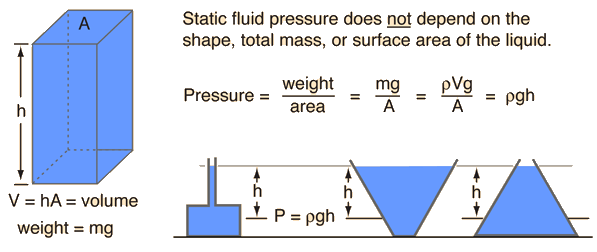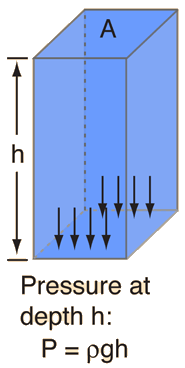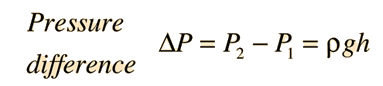# Static Fluid Pressure

The pressure exerted by a static fluid depends only upon the depth of the fluid, the density of the fluid, and the acceleration of gravity.

The pressure in a static fluid arises from the weight of the fluid and is given by the expression

 Pstatic fluid = ρgh where ρ = m/V = fluid density g = acceleration of gravity h = depth of fluid

The pressure from the weight of a column of liquid of area A and height h isThe most remarkable thing about this expression is what it does not include. The fluid pressure at a given depth does not depend upon the total mass or total volume of the liquid. The above pressure expression is easy to see for the straight, unobstructed column, but not obvious for the cases of different geometry which are shown.

Because of the ease of visualizing a column height of a known liquid, it has become common practice to state all kinds of pressures in column height units, like mmHg or cm H2O, etc. Pressures are often measured by manometers in terms of a liquid column height.

### Calculation

Index

fluid pressure calculation

Pressure concepts

 HyperPhysics***** Mechanics ***** Fluids R Nave
Go Back

# Fluid Pressure Calculation### Discussion

Fluid column height in the relationshipis often used for the measurement of pressure. After entering the relevant data, any one of the highlighted quantities below can be calculated by clicking on it.

### Pressure difference = density x g x height

If the fluid density is
ρ = gm/cm3 = kg/m3
and the column height is
h = m = x 10^ m
h = ft
then the pressure difference is
ΔP = kPa
ΔP = lb/in2
ΔP = mmHg= inches Hg
ΔP = atmos
ΔP = inches water= cm water

Note that this static fluid pressure is dependent on density and depth only; it is independent of total mass, weight, volume, etc. of the fluid.

Index

fluid pressure discussion

Pressure concepts

 HyperPhysics***** Mechanics ***** Fluids R Nave
Go Back